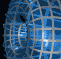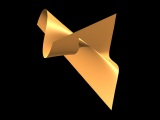Português | English Beyond 3D > Triple Point Twist > Mathematics

# The Mathematics of the Triple Point TwistThe surface shown in "Triple-Point Twist" is one from a series of surfaces described by David Mond and Washington Marar in "Real map-germs with good perturbations" , where they analyze a number of germs of singularities of surfaces. This particular example is a ruled surface given by the equations

(x,y,z) = (u, v3 + cv, uv + v5 + cv3),

where c is a parameter that can be varied. For values of c greater than 0, the surface has no self-intersection, but for values of c less than 0, a triple point and two pinch points appear. For the image shown in the exhibit, c = -1, but an MPEG movie is available showing a series of different values of c as it varies from -1 to 1. For each fixed value of u, allowing v to vary produces a planar curve in the plane x = u. The rulings for the surfaces are the straight lines produced when v is held fixed and u is allowed to vary. One of the MPEG movies shows the surface being swept out by these ruling lines.

```
```